# Fill in the Blanks¶

A Fill in the blank question can use either free text or offer options to be chosen from a drop-down list:

• Free Text Answers - Shows a question where the student must complete the missing words (fill in the blank) by entering the answers.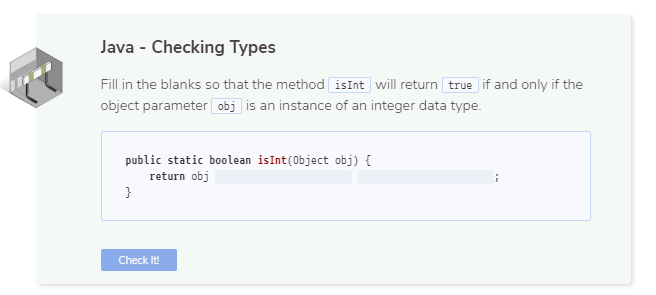• Drop-Down Answers - The possible answers are available in a drop-down list where the student chooses the correct answer.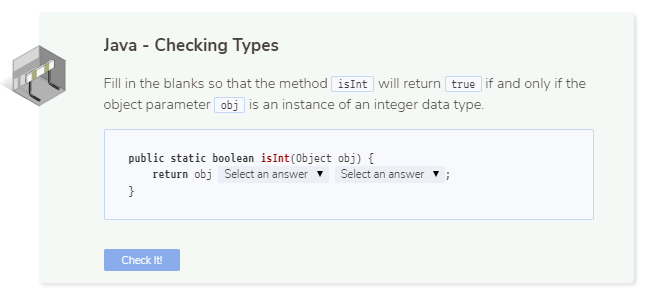Follow these steps to set up fill in the blank assessments:

1. On the General page, enter the following information: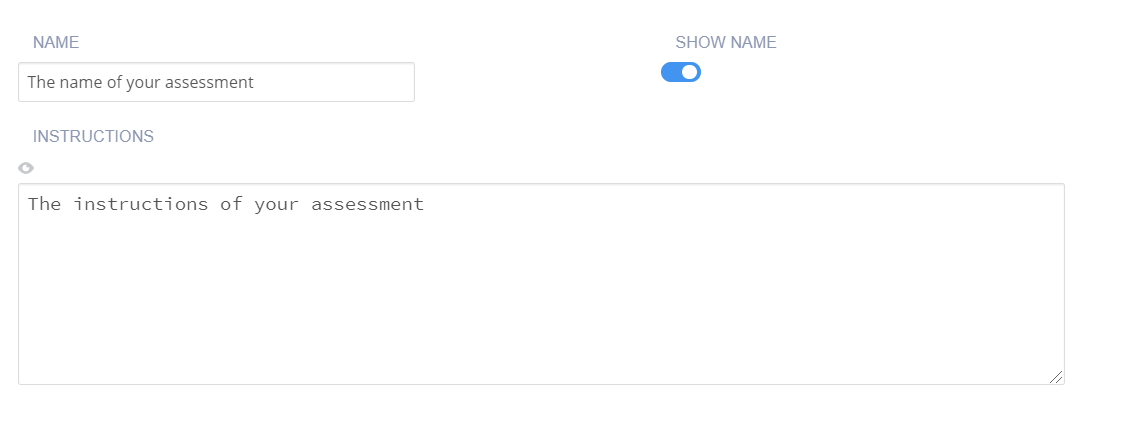• Name - Enter a short name that describes the test. This name is displayed in the teacher dashboard so the name should reflect the challenge and thereby be clear when reviewing.

Toggle the Show Name setting to hide the name in the challenge text the student sees.

• Instruction - Enter the instructions to be shown to the students.

1. Click Execution in the navigation pane and complete the following information: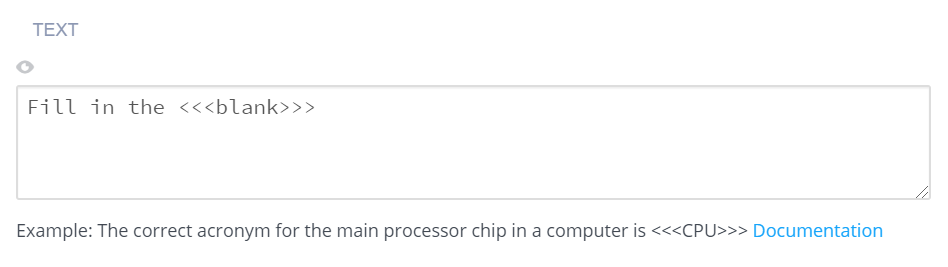• Text - Enter the question in markdown, including the correct answer specification. For example:

`A prime number (or a prime) is a <<<natural>>> number greater than <<<1>>> that has no positive divisors other than <<<1>>> and <<<itself>>>.`

• Show Possible Values - Toggle to display possible options for the correct answer:

• For text-free questions, blank fields are available for the student to enter the correct answer.

• For drop-down questions, all the correct values (anything within the <<< >>> chevrons) are provided in each of the answer positions in a randomized order. You can also add incorrect answers (one per line).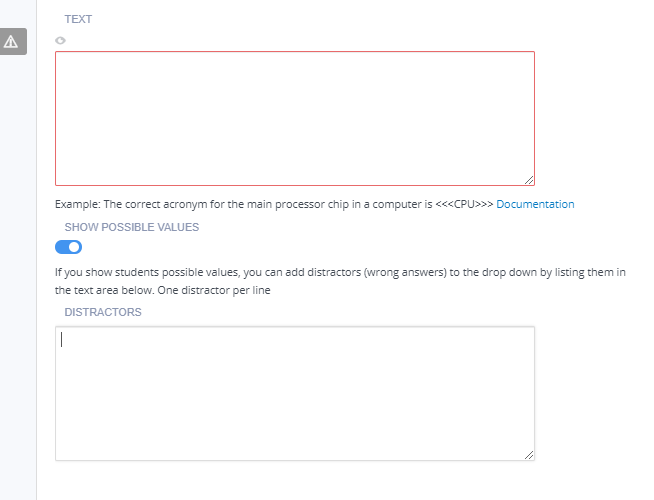Regular Expression Support

Below are some examples of regular expressions that are also supported for blank fields:

• Answer allows any characters - ``<<</./>>>``

• Answer starts with word “begin” - ``<<</^begin/>>>``

• Answer ends with word “end” - ``<<</end\$/>>>``

• Answer can contain many spaces in “this is” - `` <<</this\s+is/>>>``

• Answer contains 1 or 2 or 3 - ``<<</1 2 3/>>>``

• Answer allows color or colour - ``<<</colou?r/>>>``

• Answer allows yes or “yes” - ``<<<"yes", ""yes"">>>``

• Answer allows hat or cat - ``<<</[hc]at/>>>``

• Answer checks valid gmail address formatting - ``<<</(\W ^)[\w.\-]{0,25}@(gmail)\.com(\W \$)/>>>``

• Answer checks date format (DD/MM/YYYY) - ``<<</^(?:(?:31(\/ - \.)(?:0? 1))\1 (?:(?:29 30)(\/ - \.)(?:0?[1,3-9] 1[0-2])\2))(?:(?:1[6-9] [2-9]\d)?\d{2})\$ ^(?:29(\/ - \.)0?2\3(?:(?:(?:1[6-9] [2-9]\d)?(?:0  ) (?:(?:16  )00))))\$ ^(?:0?[1-9] 1\d 2[0-8])(\/ - \.)(?:(?:0?[1-9]) (?:1[0-2]))\4(?:(?:1[6-9] [2-9]\d)?\d{2})\$/>>>``

• Answer allows i==0 or i == 0 - ``<<</i ?== ?0/>>>``

• Answer requires digit - ``<<</^[\d]+\$/>>>``

• Answer requires non-digit - ``<<</^[\D]+\$/>>>``

• Answer requires word character - ``<<</^[\w]+\$/>>>``

• Answer requires non-word character - ``<<</^[\W]+\$/>>>``

• Answer required between 1 to 100 - ``<<</^([1-9][0-9]? 100)\$/>>>``

• Answer allows several answers (Place1 or Place2) - ``<<<"Place1", "Place2">>>``

• Answer allows several answers with/without ” ” (Place1 or “Place1” or Place2 or “Place2”) - ``<<</^(Place1 Place2 "Place1" "Place2")\$/>>>``

• Answer requires case sensitivity - ``<<</CAPITAL/>>>`` or ``<<</lowercase/>>>``

1. Click Grading in the navigation pane and complete the following fields: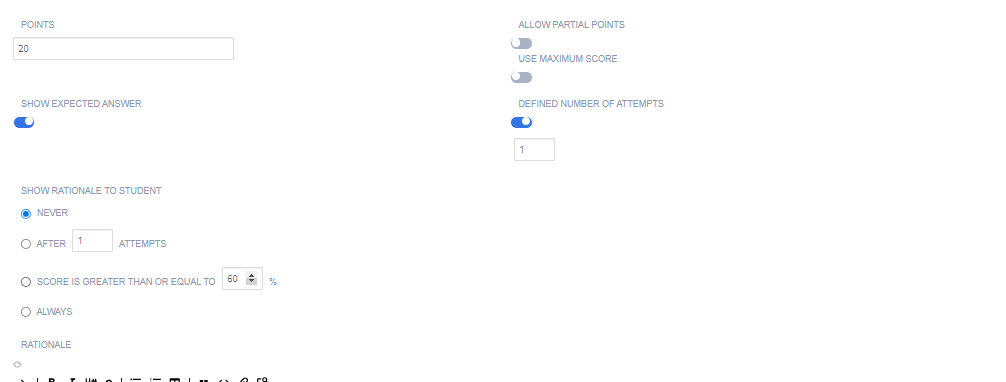• Points - Enter the score for correctly answering the question. You can choose any positive numeric value. If this is an ungraded assessment, enter zero (0).

• Show Expected Answer - Toggle to show the students the expected output when they have submitted an answer for the question. To suppress expected output, disable the setting.

• Show Answer and Rationale to Students - Toggle to display the answer, and the rationale for the answer, to the student. This guidance information will be shown to students after they have submitted their answer and any time they view the assignment after marking it as completed. You can set when to show this selecting from Never, After x attempts, If score is greater than or equal to a % of the total or Always

• Answer and Rationale - Enter guidance for the assessment. This is always visible to the teacher when the project is opened in the course or when opening the student’s project.

1. Click Metadata in the left navigation pane and complete the following fields: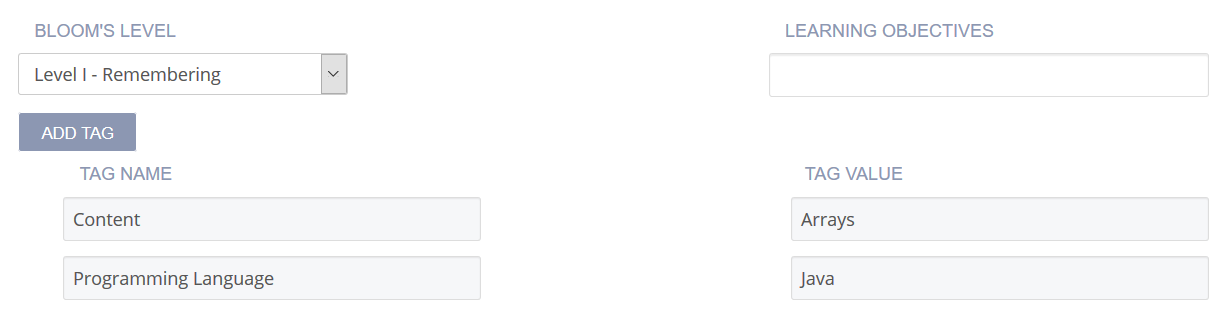• Bloom’s Level - Click the drop-down and choose the level of Bloom’s Taxonomy: https://cft.vanderbilt.edu/guides-sub-pages/blooms-taxonomy/ for the current assessement.

• Learning Objectives The objectives are the specific educational goal of the current assessment. Typically, objectives begin with Students Will Be Able To (SWBAT). For example, if an assessment asks the student to predict the output of a recursive code segment, then the Learning Objectives could be SWBAT follow the flow of recursive execution.

• Tags - The Content and Programming Language tags are provided and required. To add another tag, click Add Tag and enter the name and values.

1. Click Files in the left navigation pane and check the check boxes for additional external files to be included with the assessment. The files are then included in the Additional content list.2. Click Create to complete the process.